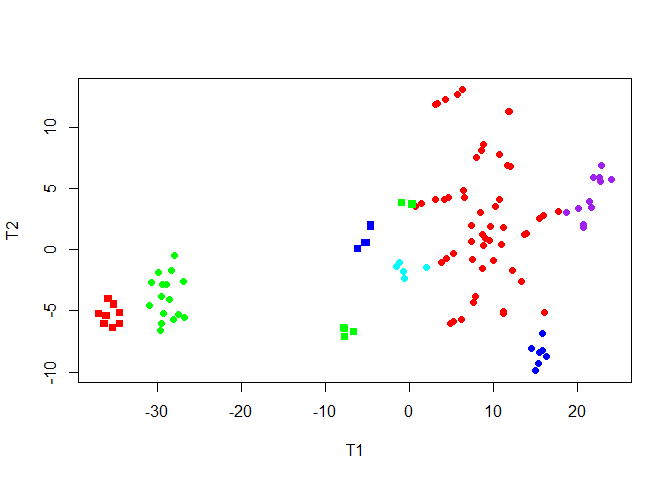# Introduction

Modern biological experiments are increasingly producing interesting binary matrices. These may represent the presence or absence of specific gene mutations, copy number variants, microRNAs, or other molecular or clinical phenomena. We have recently developed a tool, CytoGPS [^Abrams and colleagues], that converts conventional karyotypes from the standard text-based notation (the International Standard for Human Cytogenetic Nomenclature; ISCN) into a binary vector with three bits (loss, gain, or fusion) per cytoband, which we call the “LGF model”.

The Mercator package is intended to facilitate the exploration of binary data sets. It implements a subset of the 76 binary distance metrics described by [^Choi and colleagues], ensuring that at least one representative of each of their major clusters is included. Each resulting distance matrix can be combined with multiple visualiization techniques, providing a consistent interface to thoroughly explore the data set.

# The BinaryMatrix Class

suppressMessages( suppressWarnings( library(Mercator) ) )

## A Limited, Sample Dataset

We proceed with a model dataset of karyotypes from patients with Chronic Myelogenous Leukemia (CML) with 400 chromosomal features recorded over 740 patients, from the public Mitelman database. Cytogenetic abnormalities, recorded in Mitelman as text strings have been pre-processed with CytoGPS into binary vectors. For the sake of clarity and efficiency, we have chosen a subset of patients and features for this example.

filename <- system.file("Examples/Mercator_Test_Data.csv", package="Mercator")
dim(my.data)
##  740 400

## Generating the BinaryMatrix

The functions of the Mercator package operate on a BinaryMatrix S4 object, which forms the input of the subsequent functions and visualizations.

A BinaryMatrix object is formed from a matrix containing integer or numeric values. Although Mercator was designed primarily for the processing and visualization of binary data, the BinaryMatrix object and subsequent functions accept a variety of integer and numeric values.

Row and column names, along with optional annotations, can be assigned as data frames. If no row or column headings are assigned, the BinaryMatrix takes the row and column names of the inital matrix as default. Here, we wish to keep the column names associated with the parent matrix, but must create row names to build the BinaryMatrix.

Notice also that the object always includes a “history” element that tracks how it has been processed.

my.data <- as.matrix(my.data)
my.binmat <- BinaryMatrix(my.data)
summary(my.binmat)
## An object of the 'BinaryMatrix' class, of size
##  740 400
## History:
##  "Newly created."

We wish to cluster the whole karyotypes of each patient to identify the patterns of important chromosomal abnormalities that link them. To proceed, we must transpose the BinaryMatrix. Transposition meaningfully transposes the row and column headings of the BinaryMatrix, as well.

my.binmat <- t(my.binmat)
summary(my.binmat)
## An object of the 'BinaryMatrix' class, of size
##  400 740
## History:
##  "Newly created." "transposed"

## Remove Duplicate Features

The binary feature-vectors (viewed across a population of patient samples) are rarely unique. Having identical feature vectors can complicate some of the clustering and visualization routines that we want to use (often by introducing a division by zero). But they can also alter the biological implications, by automatically giving more “weight” to a single genomic event (like a trisomy or monosomy). To deal with this issue, the Mercator package includes a function to remove duplicate or redundant features.

my.binmat <- removeDuplicateFeatures(my.binmat)
summary(my.binmat)
## An object of the 'BinaryMatrix' class, of size
##  400 136
## History:
##  "Newly created."              "transposed"
##  "Duplicate features removed."

In the case of our data, only 136 of the 740 karyotypes are unique. Some of the karyotypes are “not used”, in the sense that they contain none of the abnormalities selected for this limited subset.

length(my.binmat@info$notUsed) ##  65 head(my.binmat@info$notUsed)
##  R17 R18 R23 R34 R44 R51
## 740 Levels: R1 R10 R100 R101 R102 R103 R104 R105 R106 R107 R108 ... R99

By contrast, many features are used but are simply redundant.

length(my.binmat@info\$redundant)
##  539

# Data Filtering with Thresher

Mercator provides easy access to functions of the Thresher R package, which includes outlier detection and estimates of the number of clusters [^Wang and colleagues]. The underlying idea is that the features can be viewed as “weight vectors” in a principal component space trying to display the samples. The lengths of the vectors are a measure of their importance in the data set; short vectors can (and probably should) be removed since they do not carry much useful information. We have incorporated that feature into the Mercator package.

We create a ThreshedBinaryMatrix to implement the algorithm. In general, a delta cutoff above approximately 0.3 can be chosen as standard to indicate informative feature. Then, we subset our ThreshedBinaryMatrix to only include features above our given cutoff.

set.seed(21348)
my.binmat <- threshLGF(my.binmat, cutoff=0.3)
summary(my.binmat)
## An object of the 'BinaryMatrix' class, of size
##  400 111
## History:
##  "Newly created."              "transposed"
##  "Duplicate features removed." "Threshed."

The red vertical line in the figure indicates the cutoff we have chosen to separate uninformative features (<0.3) from informative ones (>0.3).

Delta <- my.binmat@thresher@delta
hist(Delta, breaks=20, main="", xlab="Weight", col="gray")
abline(v=0.3, col='red')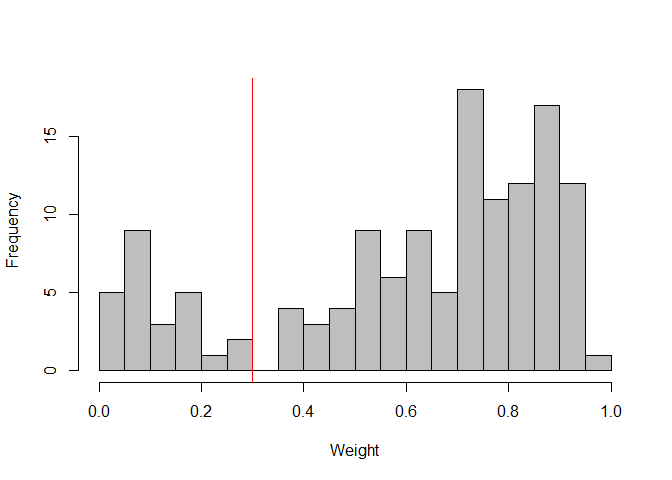Histogram of weight vectors.

The ThreshedBinaryMatrix object contains a reaper slot that estimates the number of principal components and the number of clusters after outliers have been removed. These values can be viewed numerically…

my.binmat@reaper@pcdim
##  2
my.binmat@reaper@nGroups
##  5

… or they can be visualized with an Auer-Gervini plot (where we are looking for a “long step”) …

plot(my.binmat@reaper@ag, ylim=c(0, 30))
abline(h=my.binmat@reaper@pcdim, col="forestgreen", lwd=2)
abline(h=7, col="orange", lwd=2)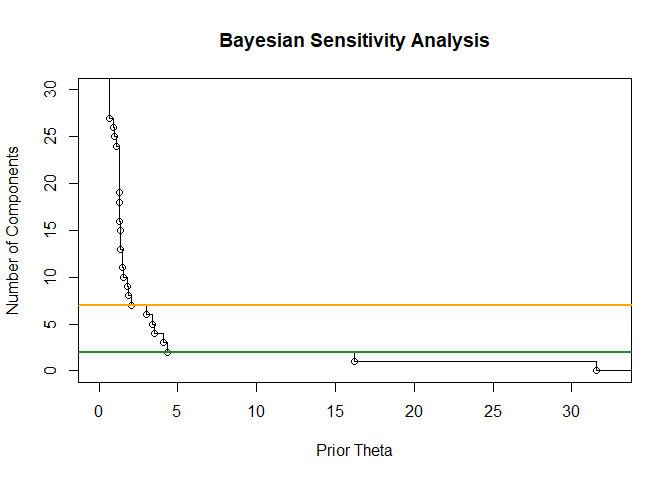Auer-Gervini plot.

pts <- screeplot(my.binmat@reaper, xlim=c(0,30))
abline(v=pts[my.binmat@reaper@pcdim], col="forestgreen", lwd=2)
abline(v=pts, col="orange", lwd=2)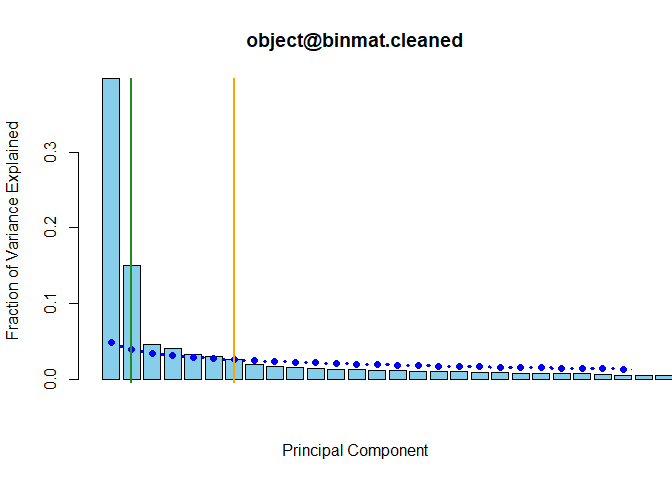Scree plot.

The default value provided by the Auer-Gervini analysis (N=2; green) is somewhat conervative. The value provided by the broken-stick model (N=7; orange) overlaid on the scree plot is more aggressive, but is not too unreasonable based on the Auer-Gervini plot. We will proceed assuming that there are seven principal components and eight clusters.

kk <- 8

# Visualization

The Mercator Package allows visualization of data with four methods, including both standard techniques (hierarchical clustering) and large-scale visualizations (multidimensional scaling (MDS), T-distributed Stochastic Neighbor Embedding (t-SNE), and iGraph.)

## Selecting a Distance Metric

The Mercator package implements or provides access to 10 distance metrics: Jaccard, Sokal-Michener, Hamming, Russell-Rao, Pearson, Goodman-Kruskal, Manhattan, Canberra, Binaryand Euclidean. Although some of these metrics can be used for continuous or categorical data, all are appropriate for some or all binary matrices. <>tt>Mercator allows the user to easily select the most appropriate metric to represent similarity and difference in a biologically meaningful way within a given dataset.

## Jaccard Distance

Here, we will use the Jaccard distance, because of its ease of interpretability, common usage, and its appropriatness of application to asymmetric binary data, such as the binary vector output of CytoGPS in this dataset.

The Mercator constructor can be called with any initial visualization, and visualizations can be added in an arbitrary order.

jacc.Vis <- Mercator(my.binmat, "jaccard", "hclust", K=kk)

We can represent all the distances between features within the dissimilarity matrix we have calculated on our data as a histogram, as a visual representation of relatedness.

hist(jacc.Vis,
xlab="Jaccard Distance", main="Histogram of Distances")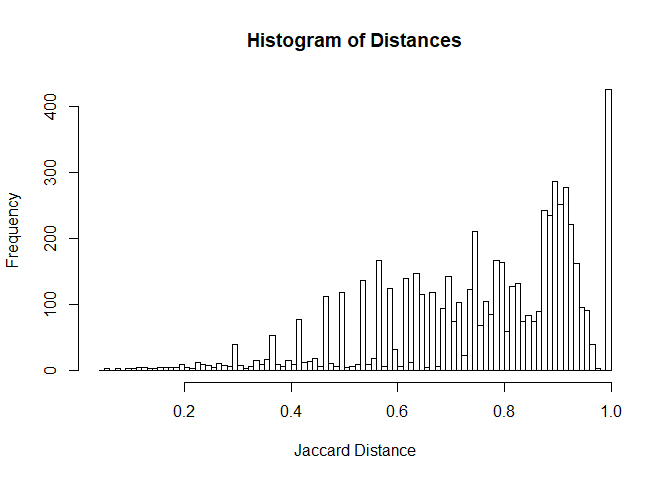Mercator allows us to implement common, standard visualizations such as hierarchical clustering…

names(jacc.Vis@view)
##  "hclust"
plot(jacc.Vis, view = "hclust")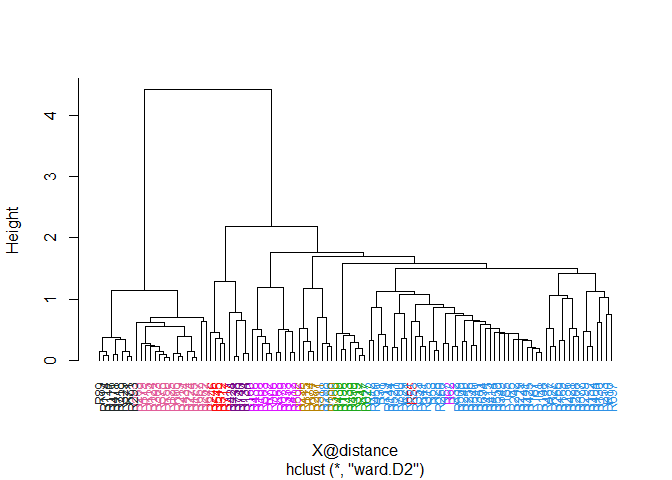Mercator can used t-distributed Stochastic Neighbor Embedding (t-SNE) plots for visualizing large-scale, high-dimensional data in 2-dimensional space.

par(pty="s")
perplexity=5,
xlab="T1", ylab="T2")
names(jacc.Vis@view)
##  "hclust" "tsne"
plot(jacc.Vis, view = "tsne", main="t-SNE; Jaccard Distance; perplexity=5")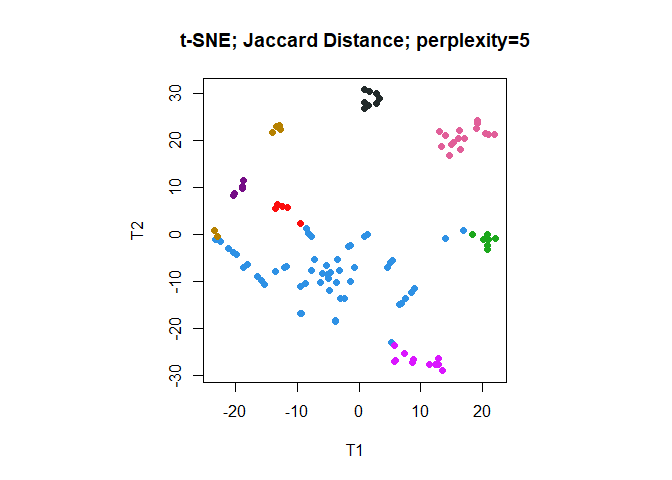Optional t-SNE parameters, such as perplexity, can be used to fine-tune the plot as the visualization is created. Using addVisualization to create a new, tuned plot of an existing type overwrites the existing plot of that type.

jacc.Vis <- addVisualization(jacc.Vis, "tsne",
perplexity=10)
## Warning in addVisualization(jacc.Vis, "tsne", perplexity = 10): Overwriting
## an existing visualization:tsne
names(jacc.Vis@view)
##  "hclust" "tsne"
plot(jacc.Vis, view = "tsne",  main="t-SNE; Jaccard Distance; perplexity=10")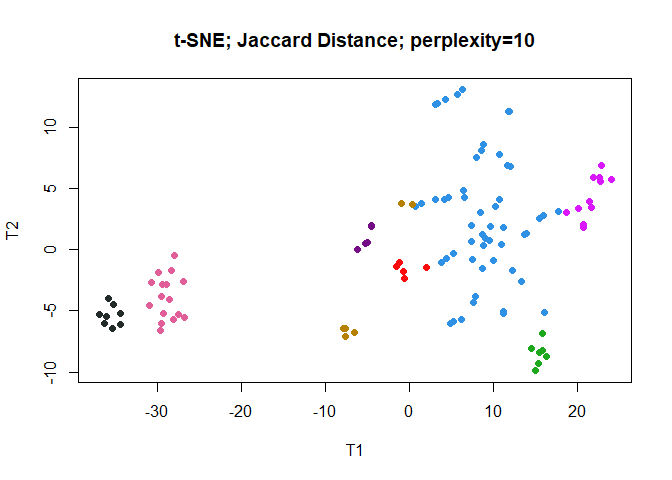par(pty="m")

Mercator allows visualization of multi-dimensional scaling (MDS) plots, as well.

jacc.Vis <- addVisualization(jacc.Vis, "mds")
names(jacc.Vis@view)
##  "hclust" "tsne"   "mds"
plot(jacc.Vis, view = "mds", main="MDS; Jaccard Distance")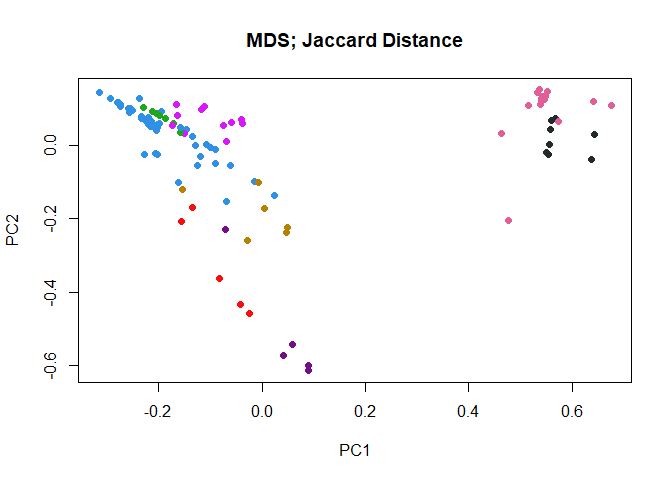### iGraph

Mercator can be used to visualize complex networks using iGraph. To improve clarity of the visualization and computational time, we implement the downsample function to reduce the number of data points to be linked and visualized. The idea goes back to Peng Qiu’s implementation of the SPADE clustering algorithm for mass cytyometry data. The main point is to under sample the densest regions of the data space to make it more likely that rarer clusters will still be adequately sampled. (While not required with the current data set, this idea can be quite useful with data sets containng tens of thousands of objects.)

Note: The Mercator class includes a “subset” operator that tries to preserve earlier visualizations. This operator is fast for MDS or t-SNE models, but is very slow for large hierarchical clustering. (It uses the implementation in the dendextend package, which works by removing a single leaf at a time from the tree.) In the next code chunk, we first throw away the dendrogram, then subset using the downsampled data, and then compute a new dendrogram.

X <- jacc.Vis
N <- as.matrix(X@distance)
set.seed(87530)
P <- downsample(40, N, 0.1)
J <- jacc.Vis[P]
J <- addVisualization(J, "tsne", perplexity=5)
## Warning in addVisualization(J, "tsne", perplexity = 5): Overwriting an
## existing visualization:tsne
names(J@view)
##  "hclust" "tsne"   "mds"
par(pty="s")
plot(J, view = "tsne", main="Down-sampled t-SNE Plot")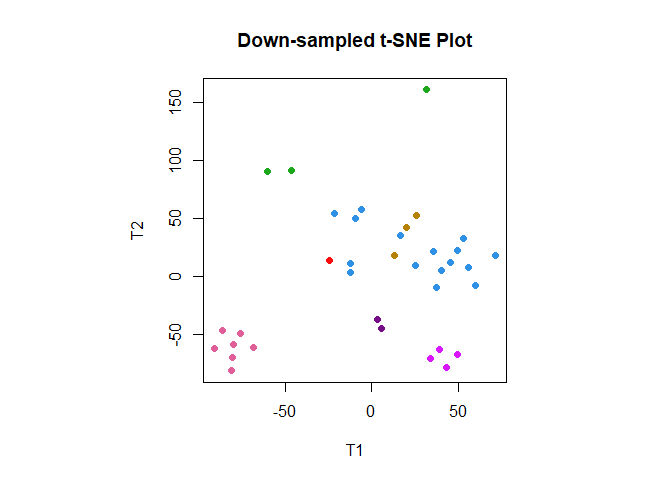par(pty="m")

The densest “eyes” are heavily under-sampled.

Now we can look at the resulting graph, using three different “layouts”.

set.seed(10967)
J <- addVisualization(J, "mds")
## Warning in addVisualization(J, "mds"): Overwriting an existing
## visualization:mds
J <- addVisualization(J, "graph")

plot(J, view = "graph", layout = "mds")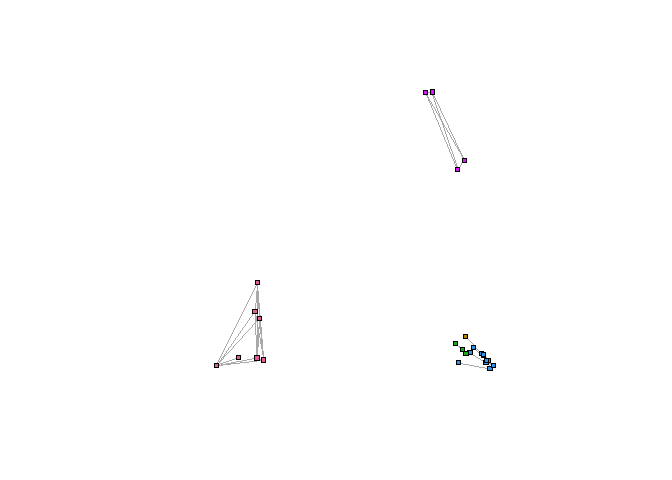plot(J, view = "graph", layout = "nicely",
main="Graphical View of Down-sampled Jaccard Distance Matrix",
xlim=c(-1,1))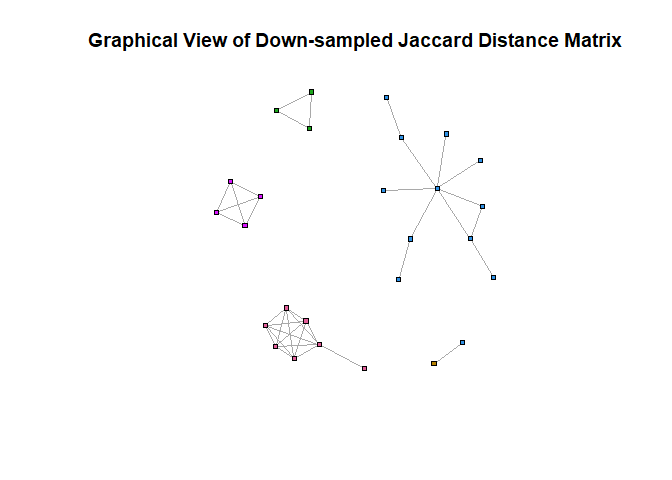plot(J, view = "graph", layout = "tsne", main="T-SNE Layout")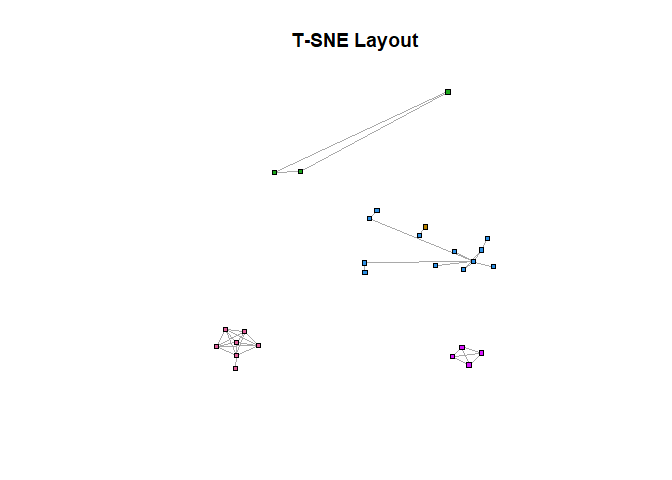# Cluster Identities

We can use the getClusters function to characterize each cluster and use these for further manipulation.

We can easily determine cluster size…

my.clust <- getClusters(jacc.Vis)
tab <- table(my.clust)
tab
## my.clust
##  1  2  3  4  5  6  7  8
## 53 16  7  5 11  8  6  5

… or the patients that comprise each cluster.

C <- my.binmat@columnInfo
Cl4 <- C[my.clust == 4 ,]
Cl4
##  R55  R246 R312 R545 R717
## 740 Levels: R1 R10 R100 R101 R102 R103 R104 R105 R106 R107 R108 ... R99

# Tuning

Finally, we are going to look at what happens if we use a different distance metric.

set.seed(8642)
sokal.Vis <- Mercator(my.binmat, "sokal", "tsne", K=kk, peplexity = 10)
table(getClusters(sokal.Vis), getClusters(jacc.Vis))
##
##      1  2  3  4  5  6  7  8
##   1 53  0  7  5  4  0  2  1
##   2  0 15  0  0  0  0  0  0
##   3  0  0  0  0  0  8  0  0
##   4  0  0  0  0  7  0  0  0
##   5  0  0  0  0  0  0  4  0
##   6  0  0  0  0  0  0  0  2
##   7  0  1  0  0  0  0  0  0
##   8  0  0  0  0  0  0  0  2
plot(sokal.Vis, view = "tsne", main="t-SNE; Sokal-Michener Distance; perplexity=10")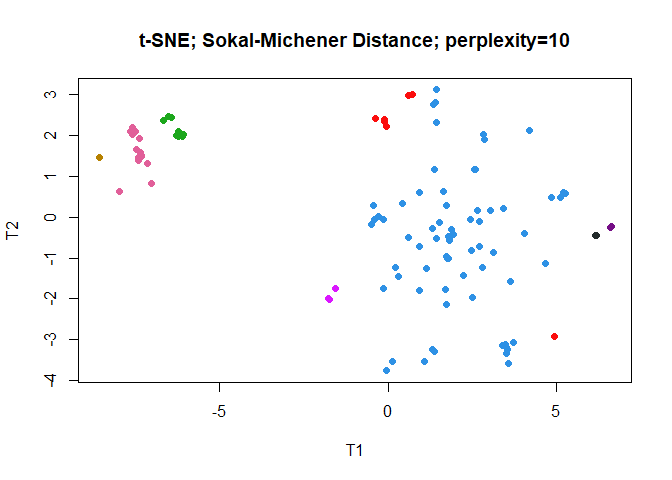The two larges groups get assigned the same colors (by chance) in the Jaccard and the Sokal-Michener clusterings. However, it is not at all clear how to align the smaller groups. For that purpose, we can use the remapColors function.

SV <- remapColors(jacc.Vis, sokal.Vis)
table(getClusters(SV), getClusters(jacc.Vis))
##
##      1  2  3  4  5  6  7  8
##   1 53  0  7  5  4  0  2  1
##   2  0 15  0  0  0  0  0  0
##   3  0  1  0  0  0  0  0  0
##   4  0  0  0  0  0  0  0  2
##   5  0  0  0  0  7  0  0  0
##   6  0  0  0  0  0  8  0  0
##   7  0  0  0  0  0  0  4  0
##   8  0  0  0  0  0  0  0  2
plot(SV, view = "tsne", main="t-SNE; Sokal-Michener Distance; perplexity=10")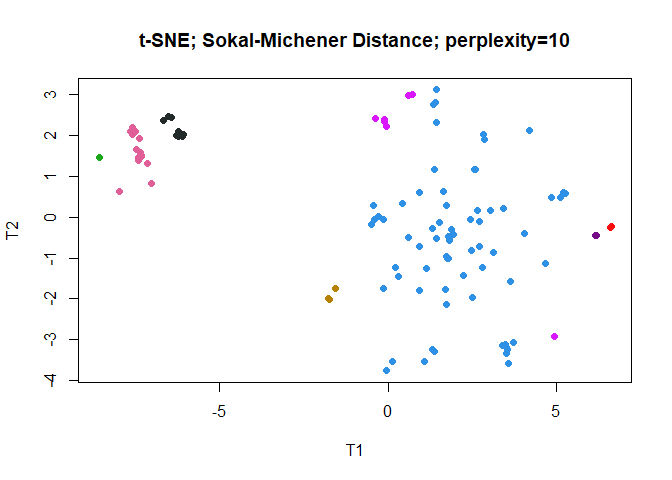Now colors have been matched (as well as possible) between the two sets of visualizations.

# Changing the Color Palette

Each Mercator object stores its own palette internally, but you can change the palette using the slot funciton.

slot(jacc.Vis, "palette") <- c("red", "orange", "green", "blue",
"cyan", "magenta", "purple", "black")
plot(jacc.Vis, view = "tsne")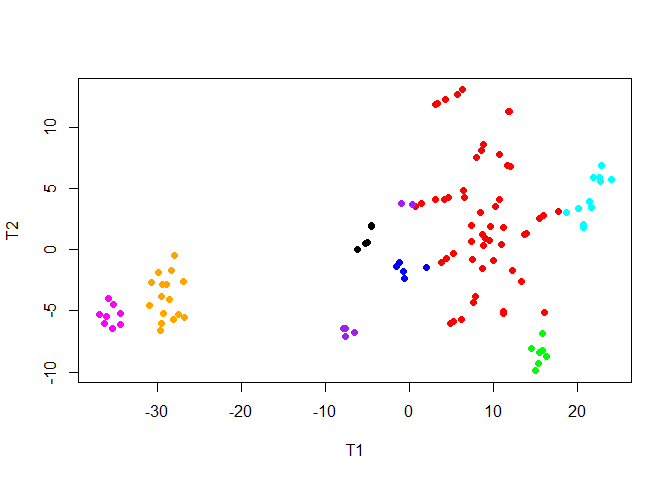If the number of colors in the palette is smaller thant he numbe of clusters, they will be recycled, and other plotting symbols will be introduced.

slot(jacc.Vis, "palette") <- c("red", "green", "blue",
"cyan", "purple")
plot(jacc.Vis, view = "tsne")